# Accompanying Math for Sample Problems

To accompany the sample problems that appear on this website I am working on creating some math background information. This is not area that I am an expert but I have been working towards improvement.

Several of the sample problems require the use of trigonometric functions or “trig” for short. Understanding what a “function” is a good first step.  In a function there is an input variable and an output variable that is the result of some process performed on the input variable. For a common basic trig function the input will be an angle in degrees and the output will be a decimal number.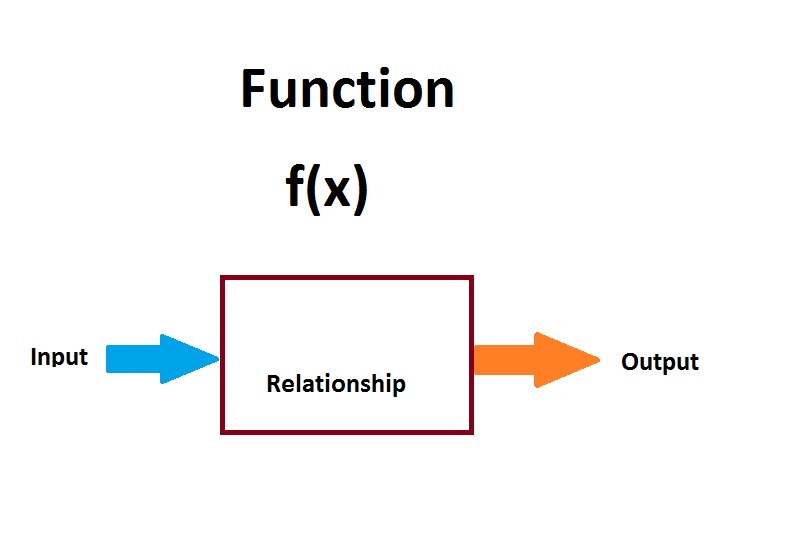Example: 30 degrees input into sine function and output is .5

It is Important to Know the Sides of a Right Triangle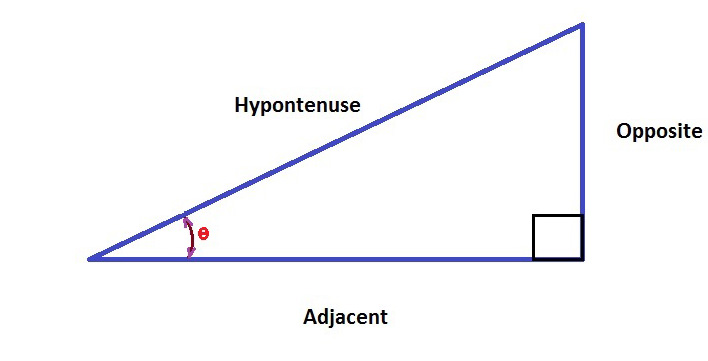More Commonly Used Trig Functions    Abbreviated

Sine = Opposite / Hypotenuse                       sin
Cosine = Adjacent / Hypotenuse                  cos
Tangent = Opposite / Adjacent                       tan

You will notice the additional three trig functions have the fractions created by the sides inverted which is known as the “reciprocal”.

Cosecant = Hypotenuse / Opposite           csc
Secant = Hypotenuse / Adjacent                 sec
Cotangent = Adjacent / Hypotenuse         cot

Finding the Function Values

In the past people might look up trig values in tables or use a scientific calculator. The Windows operating system includes a calculator that can provide the functions when viewed in scientific mode.Calculator that is part of Windows operating system found under Accessories.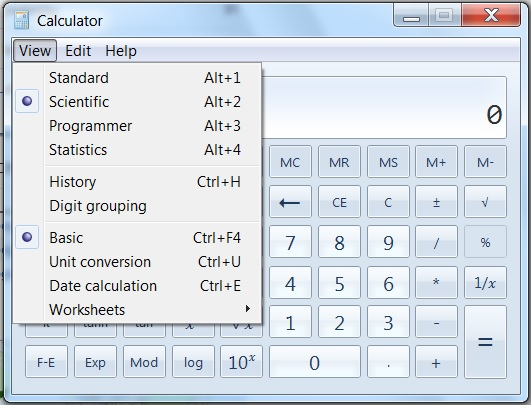Clicking on the View menu and selecting Scientific will give trig functions.

Applying a Function to a Sample ProblemLift = Weight x Cosine Angle of Climb

Lift = 10 grams x cosine(30)

Lift = 10 grams x .866

Lift = 8.66 grams

Inverse Trig Functions

Another corresponding set of functions are available that use the value of two sides and output the corresponding angle. The input is a fraction created by the values of the two sides.

These functions are known as the inverse trig functions the confusing part is that the notation may differ.  The functions may be shown like the regular trig functions with a -1 superscript; example tan-1      or with an “arc” prefix.  Using the arc avoids the issue of confusing with exponents.

The Inverse Functions

Arcsine    arccosine   arctangent
Arccotangent  arcsecant arccosecant

This is an example of using the arctangent function in an example from Basic Aerodynamics With a Lesson:

Glide Angle = (Height / Distance) Arctangent

Calculate the glide angle if launched from a height of 1 meter and glider travels over 5 meter distance?

I will demonstrate how to use the Windows Calculator to use the Arctangent function.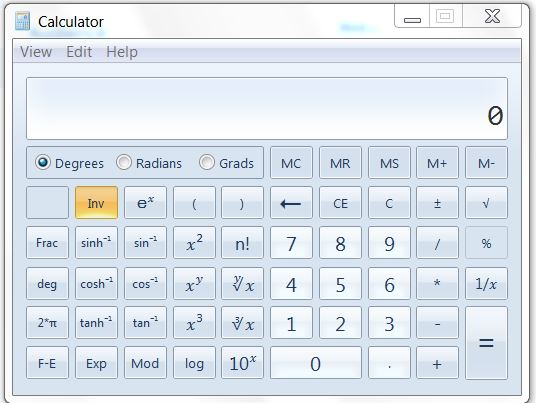Pressing the Inv key will convert the trig function keys to the corresponding inverse trig function.

Glide Angle = (1 meter / 5 meter) arctangent

Glide Angle = .2 x arctangent

Glide Angle = 11.3 degrees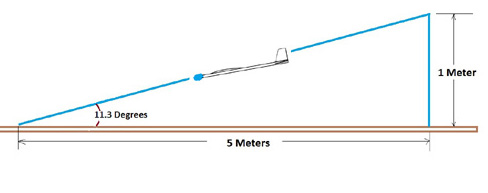It is interesting to check this in reverse using the 11.3 degree glide angle and the travel distance of 5 meters.

Height = tan(11.3) x 5
Height = 1 meter when rounded

## One thought on “Accompanying Math for Sample Problems”

1.Anonymous says:

Have fun experimenting and keep me posted on the adventure!Looks amazing! Will have to take this on when I have some spare time